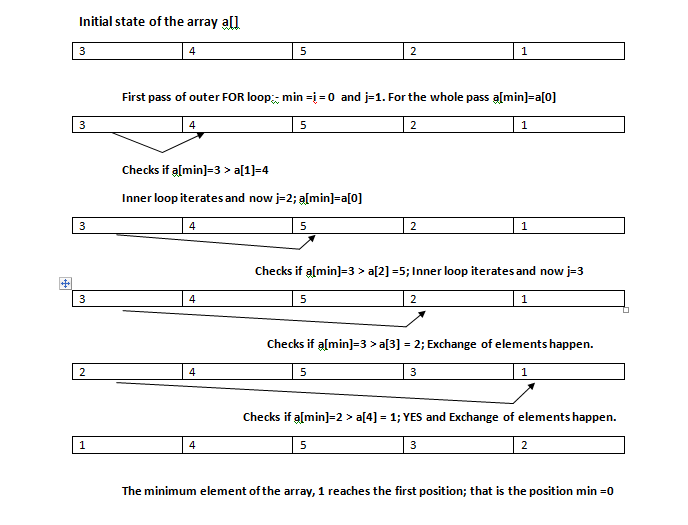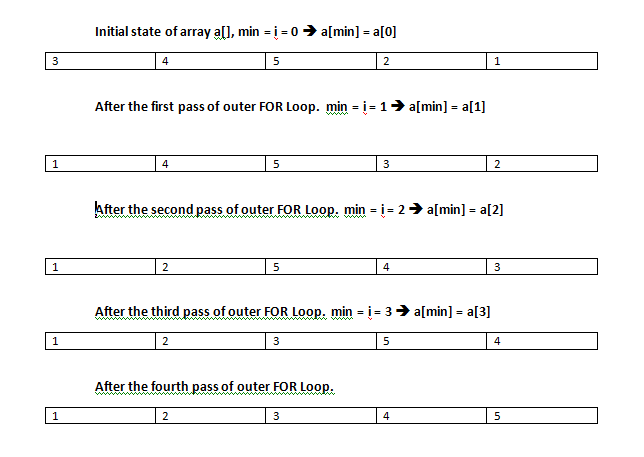## Audio

[Audio][slideshow]

In the previous article, we have analysed Bubble sort algorithm and it’s implementation in C programs. In this article we are going to see another sorting algorithm, named, Selection sorting. Just like “Bubble sort”, selection sort is also considered as an inefficient sorting algorithm. If you are curious to know the reason for this, please read the article about Bubble sort carefully. The number of comparisons involved in an average bubble sort is 0.5*(n*n-n). Same is the index for Selection sort too.

Note:- Since the algorithm is implemented with the help of 2 FOR loops only, it can be used as such for any programming languages like C/C++ or Java

Let’s get into algorithm analysis. Consider an array of the order 3, 4, 5, 1, 2. Let’s sort this array in ascending order using selection sort algorithm. Bubble sort algorithm was based on repeated comparison of successive elements in an array and exchanging elements if necessary. In selection sort there is a slight difference. One element in the array (usually the first/last element) is assumed as the minimum/maximum of the whole array elements. Now all other members are compared against this assumed min/max element. Based on this comparisons result, exchanges are made between the min/max element and the other element.

Lets study this algorithm through an example. The code snippet for implementing selection sort in C is given below. The code performs sorting in ascending order using selection sort.

`/* limit = number of elements in the array *//* min = variable used to hold the assumed minimum element */`

for(i=0; ia[j]) // Compares the minimum element with all other members using inner FOR loop.
{
temp=a[j]; // 3 statements below exchanges the elements
a[j]=a[min];
a[min]=temp;
}
}
}

Please see the 2 images given below.All the four passes of outer FOR loop is shown in the image below:-#### Working of Selection sorting

You can easily understand the working of selection sort by interpreting the 2 images given above & the example code snippet. The element in the first position is assumed to be minimum. It is then compared with other elements one by one (using the 2nd FOR loop). After the first pass of the first FOR loop, the minimum of the whole array (in this case 1) is placed in first position of the array. When the second pass of first FOR loop begins, the next element (i.e second element) is assumed to be minimum and the whole process repeats.

The selection sorting algorithm is also an inefficient one (just like Bubble sort). Selection sort requires 0.5 *(n*n-n) comparisons. The total number of comparisons required is almost same for both Bubble sorting and Selection sorting. However the total number of exchanges required (in the average case) is very low for selection sorting. We can assume that in an average case, Selection sort is much efficient than Bubble sorting algorithm.

Just like we made the Bubble sort algorithm better with slight modifications, we can make the Selection sort algorithm better too. You may go through the Bubble Sorting in C/C++ article and find out how modifications are made in the algorithm. Think how can we modify selection sort algorithm for better performance.

[Lights][grids]